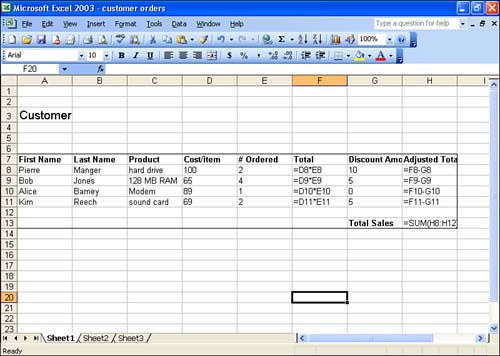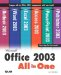# Understanding Excel Formulas

One way to add calculations to an Excel workbook is to create your own formulas. Formulas are typically used to perform calculations such as addition, subtraction, multiplication, and division. More complex calculations are better left to Excel functions, which is a built-in set of formulas that provide financial, mathematical, and statistical calculations. You learn more about functions in Lesson 5, "Performing Calculations with Functions."

Formulas that you create typically include cell addresses that reference cells on which you want to perform a calculation. Formulas also consist of mathematical operators, such as + (addition) or * (multiplication). For example, if you wanted to multiply two cells , such as C3 and D3, and then divide the product by 3, you would design a formula that looks like this:

` =(C3*D3)/3 `

Notice that the formula begins with the equal sign (=). This lets Excel know that the information that you are placing in the cell is meant to do a calculation. The parentheses are used to let Excel know that you want C3 multiplied by D3 before the result is divided by 3. Creating appropriate formulas requires an understanding of the order of mathematical operations, or what is often called the rules of precedence. The natural order of math operations is covered in the next section.

#### Formula Operators

As previously mentioned, you can create formulas that add, subtract, and multiply cells in the worksheet. Table 3.1 lists some of the operators that you can use and how you would use them in a simple formula.

##### Table 3.1. Excel's Mathematical Operators

Operator

Performs

Sample Formula

Result

^

Exponentiation

=A1^3

Enters the result of raising the value in cell A1 to the third power

+

Addition

=A1+A2

Enters the total of the values in cells A1 and A2

Subtraction

=A1A2

Subtracts the value in cell A2 from the value in cell A1

*

Multiplication

=A2*A3

Multiplies the value in cell A2 by cell A3

/

Division

=A1/B1

Divides the value in cell A1 by the value in cell B1

Figure 3.1 shows some formulas that have been created for an Excel worksheet. So that you can see how I wrote the formulas, I've configured Excel so that it shows the formula that has been placed in a cell rather than the results of the formula (which is what you would normally see).

##### Figure 3.1. You can create formulas to do simple calculations in your worksheets.#### Order of Operations

The order of operations, or operator precedence , simply means that some operations take precedence over other operations in a formula. For example, in the formula =C2+D2*E2, the multiplication of D2 times E2 takes precedence, so D2 is multiplied by E2 and then the value in cell C2 is added to the result.

You can force the precedence of an operation by using parentheses. For example, if you want C2 and D2 added before they are multiplied by E2, the formula would have to be written =(C2+D2)*E2.

The natural order of math operators follows :

1. Exponent (^) and calculations within parentheses

2. Multiplication (*) and division (/)

3. Addition (+) and subtraction ()

In the case of operations such as multiplication and division, which operate at the same level in the natural order, a formula containing the multiplication operator followed by the division operator will execute these operators in the order they appear in the formula from left to right. If you don't take this order into consideration, you could run into problems when entering your formulas. For example, if you want to determine the average of the values in cells A1, B1, and C1, and you enter =A1+B1+C1/3 , you'll get the wrong answer. The value in C1 will be divided by 3, and that result will be added to A1+B1. To determine the total of A1 through C1 first, you must enclose that group of values in parentheses: =(A1+B1+C1)/3 .Microsoft Office 2003 All-in-One
ISBN: B005HKSHB2
EAN: N/A
Year: 2002
Pages: 660
Authors: Joe Habraken

Similar book on Amazon

flylib.com © 2008-2017.
If you may any questions please contact us: flylib@qtcs.net